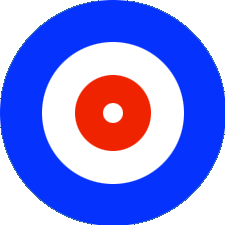SEARCH HOMEMath Central Quandaries & QueriesQuestion from Fatima, a parent: In the olympic event of curling, the scoring area consists of four concentric circles on the ice with radii of 6 inches, 3 feet, 4 feet, and 6 feet If a team member lands a (43 pound) stone randomly within the scoring area, find the probability that it ends up centred on a.red b. white c.blue I am explaining the figure also in words The smallest Circle is of White color then a blue colour circle then again a White colour circle then the last big circle of red color The answer for a bit is 5/9 I tried it with many ways but I am not getting the answer Please clearly tell me what is n(S)and what is n(E). QuestionI have drawn the rings for the game of curling.I am going to calculate the stone ends up un the blue ring.

First of all you need to assume that the curling stone is a point at a random position in the rings. The probability that it is in the blue ring is the fraction of the area of the rings that is blue.

The region painted blue is the region inside a circle of radius 6 feet and outside the circle with the same center but with radius 4 feet. The area inside a circle or radius r feet is $\pi r^2$ and hence the area inside a circle of radius 6 feet is $\pi 6^2$ square feet. The area inside a circle of radius 4 feet is $\pi 4^2$ square feet. Thus the area of the region painted blue is

$6^2 \pi- 4^2 \pi = (36 - 16) \pi = 20 \pi \mbox{ square feet.}$

Thus the probability that the rock is in the blue ring is

$\frac{20 \pi}{36 \pi} = \frac59$

Now you try the other colours.

PennyMath Central is supported by the University of Regina and The Pacific Institute for the Mathematical Sciences.